# 水位变化对正倒锥体冰载荷影响的离散元分析1)

*大连理工大学工业装备结构分析国家重点实验室,大连 116024

# INFLUENCE OF WATER LEVEL ON ICE LOAD ON UPWARD-DOWNWARD CONICAL STRUCTURE BASED ON DEM ANALYSIS1)

Long Xue*, Liu Shewen, Ji Shunying*2)*State Key Laboratory of Structure Analysis of Industrial Equipment, Dalian University of Technology, Dalian 116024, China
Nava Architecture and Engineering College,Dalian Maritime University, Dalian 116026, China

Abstract

During the interaction between the sea ice and the conical structure, the ice load is affected by the position of the sea ice acting on the conical structure when the tidal water level changes. In this study, the discrete element method (DEM) with the bond and failure model is adopted to simulate the breaking process of sea ice acting on the conical structure. In DEM simulations, the influence of the ice temperature at the top and bottom surface of ice cover on the ice strength is considered. The ice load and the failure mode of ice cover simulated with DEM are compared well with the field data in the Bohai Sea. The DEM results indicate that the bending failure of ice cover occurs when it acts on the upward or downward cone. For both of the upward and downward cone, the ice load increases with the increase of the cone diameter at water level, but the ice load on upward cone is larger than that of downward cone. Meanwhile, the breaking length of ice cover on upward cone is smaller than that on the downward cone. The reasons for the difference of ice load and breaking length between upward cone and downward cone are analyzed based on the DEM results and the filed observation in the Bohai Sea. Moreover, when the sea ice interacts with the interface of upward and downward cone, bending damage generally occurs. When the height of the ice cover center is very close to the height of the upward-downward cone interface, the local ice crushing occurs, but the ice load does not increase significantly. Therefore, the downward conical structure has better performance of ice resistance with effectively reducing the ice load. The DEM can be adopted to understand the mechanism of ice cover failure process, and provide reference for the anti-ice design of structures in cold ocean engineering.

Keywords： discrete element method ; conical structure ; ice load ; failure mode ; interface of upward and downward cone

0

Long Xue, Liu Shewen, Ji Shunying. INFLUENCE OF WATER LEVEL ON ICE LOAD ON UPWARD-DOWNWARD CONICAL STRUCTURE BASED ON DEM ANALYSIS1)[J]. Chinese Journal of Theoretical and Applied Mechanics, 2019, 51(1): 74-84 https://doi.org/10.6052/0459-1879-18-342

## 1 海冰与锥体结构作用的离散元方法

### 1.1 海冰颗粒单元的平行粘结模型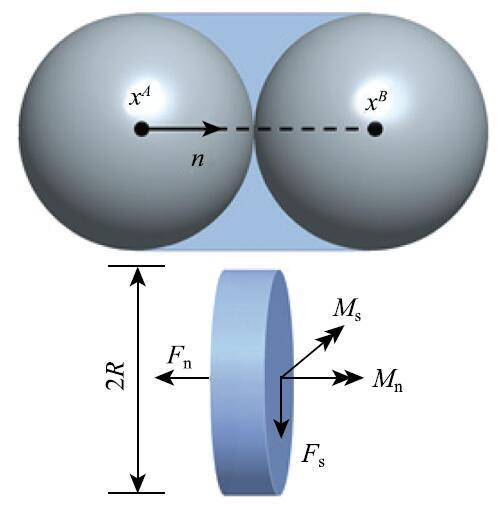Fig. 1   Parallel bond method of spherical particles

\begin{equation}\label{eq1} \sigma _{\max } = \frac{ F_{n} }{A} + {sign}(F_{n}) \frac{\left| {M_{s} } \right|}{I}R, \quad\tau _{\max } = \frac{\left| {F_{s} } \right|}{A} +\frac{\left| {M_{n} } \right|}{J}R \tag{1}\end{equation}

\begin{equation}\label{eq2} A = \pi R^2, \quad I = \frac{1}{4}\pi R^4, \quad J= \frac{1}{2}\pi R^4 \tag{2}\end{equation}

### 1.2 海冰粘结颗粒单元的失效准则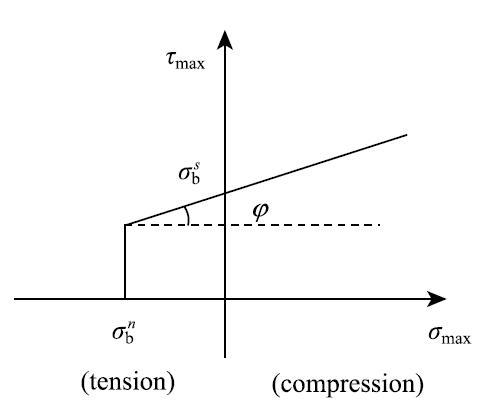Fig.2   Failure criterion of bonded particles

$$\sigma ^{t} = \sigma _{b}^{n} \tag{3}$$

$$\tau ^{s} = \sigma _{b}^{s} + \mu _{b} \sigma_{\max } \tag{4}$$

### 1.3 海冰强度与温盐分布的对应关系

$$v_{b} = S_{i} \left(0.532 + \frac{49.185}{\left|{T_{i} } \right|}\right) \tag{5}$$

$$\sigma _{f} = 2.98{e}^{-5.06\sqrt {v_{b} } } \tag{6}$$

\begin{equation}\label{eq7} \sigma _{b} = \sigma _{f} / (1.77-1.81{e}^{-\frac{h_{i} / D_{p} }{4.78}})\tag{7}\end{equation}

## 2 海冰与正倒锥结构作用的离散元分析}

### 2.1 海冰离散元参数的设定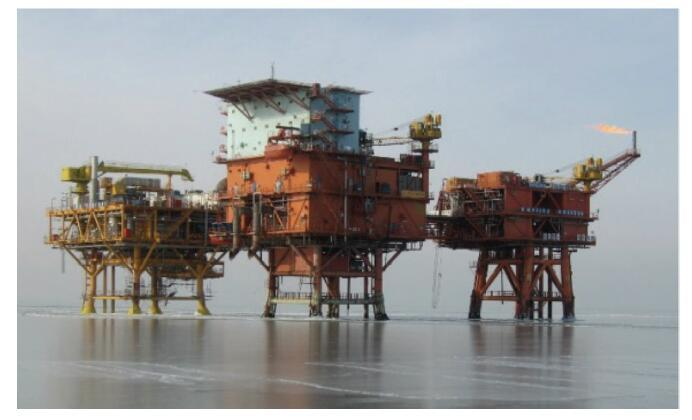Fig.3   The JZ20-2 jacket platform with upward-downward\ cone in the Bohai Sea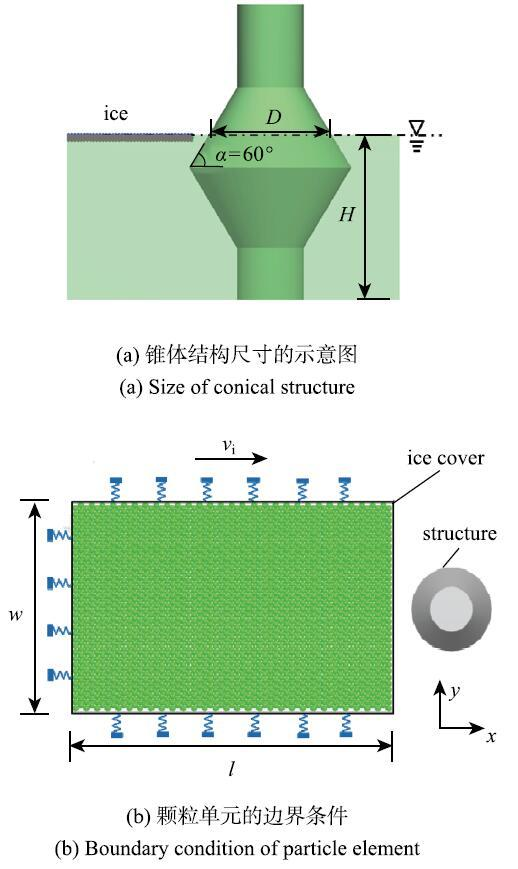Fig.4   DEM of the interaction between ice and conical structure

Table 1   Main parameters of DEM calculation

DefinitionSymbolValue
density of sea waterPw1035.0 kg/m3
density of sea icePi920.0 kg/m3
velocity of sea iceVi0.4 m/s
thickness of sea iceh0.23 m
calculation area size of sea icel x w13.0 mx13.0 m
elastic modulus of sea iceE1.0 GPa
diamete of particle celldp0.054 m
total number of particle elementsN2.9x105
friction coefficient of particle element0.2
coefficient of friction between sea ice and structure0.2
salinity of sea iceS i1.0 %〇
upper and lower surface temperatures of sea iceT-7.0°C,-1.73°C

### 2.2 正锥与倒锥上冰载荷的对比分析

(1) 正锥体上的冰载荷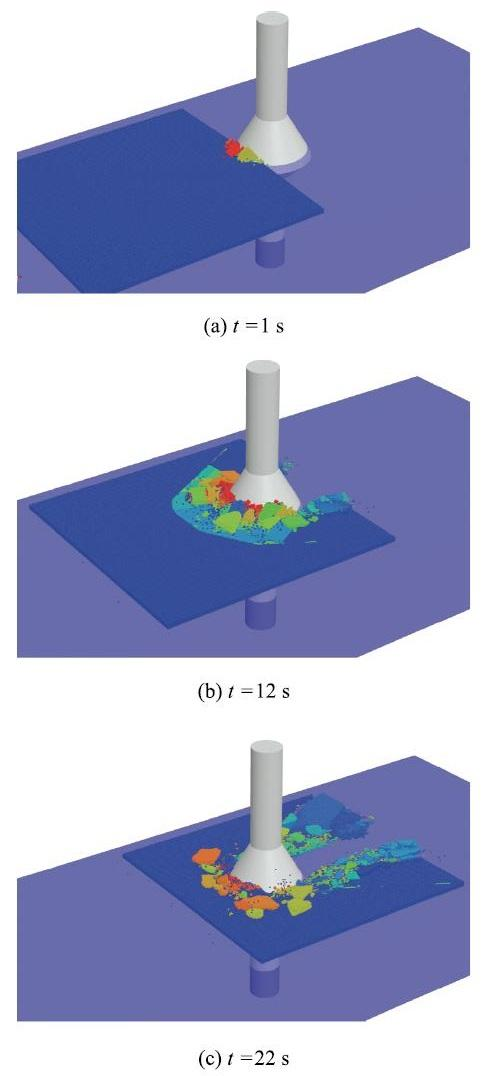Fig.5   Failure process of sea ice acting on upward cone simulated by DEM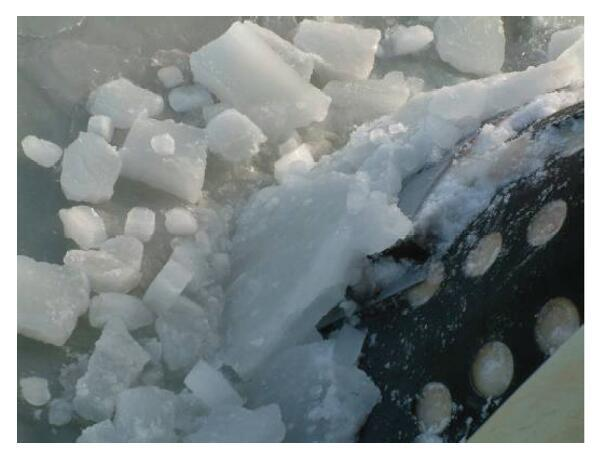Fig.6   Interaction of sea ice and upward cone observed on the JZ20-2 platform in the Bohai Sea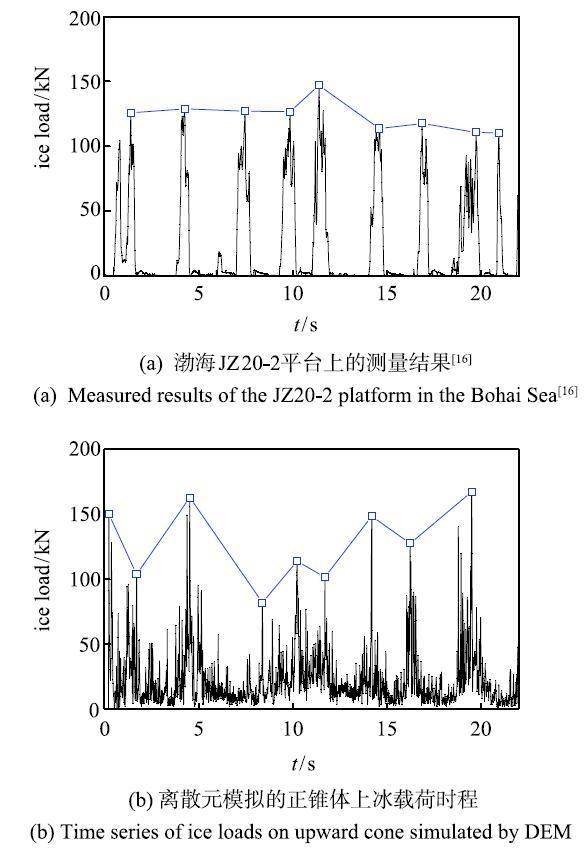Fig.7   Comparison of ice loads on upward cone measured in the Bohai Sea and simulated with DEM

(2) 倒锥体上的冰载荷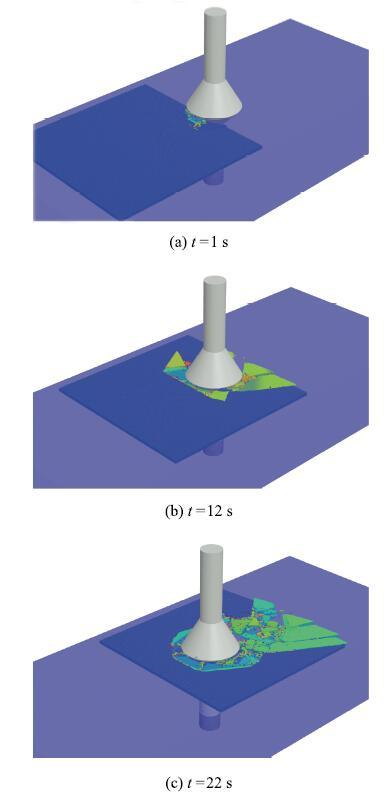Fig.8   Breaking process of sea ice acting on downward cone simulated with DEM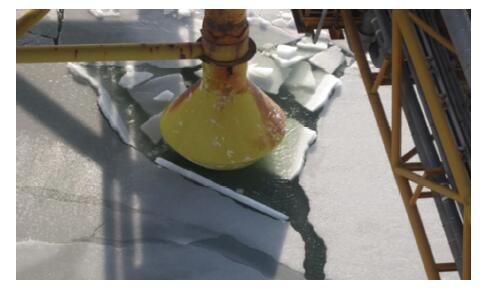Fig.9   Interaction of sea ice and downward cone observed the JZ20-2 platform in the Bohai Sea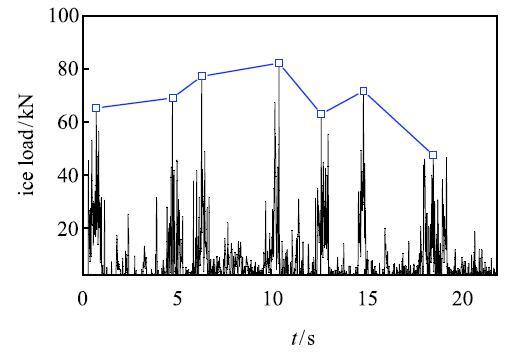Fig.10   Time series of ice loads on downward cone simulated by DEM

### 2.3 正锥与倒锥上海冰断裂长度的对比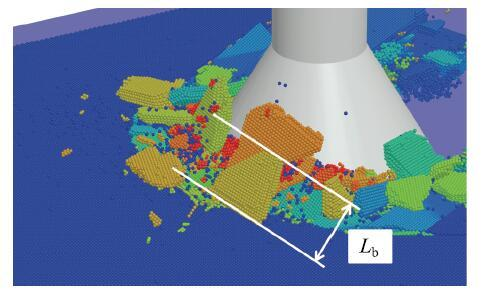Fig.11   Breaking length of ice cover simulated with DEM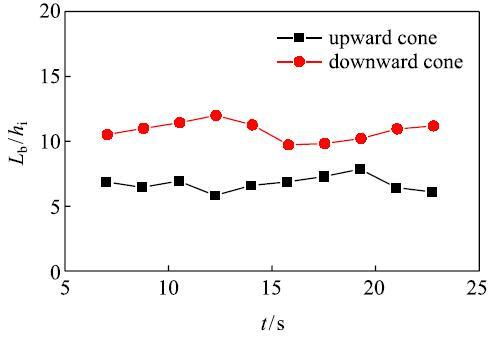Fig.12   Breaking length of ice cover acting on upward and\ downward cones

## 3 海冰与正倒锥作用位置对冰载荷的影响

### 3.1 锥径影响下正锥和倒锥体上的冰载荷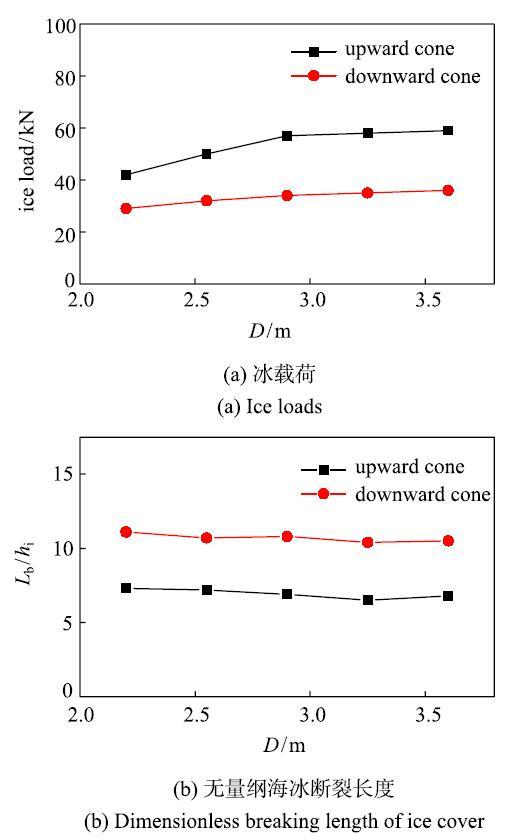Fig.13   Effect of cone diameter on ice load and breaking length

### 3.2 正倒锥交界处的冰载荷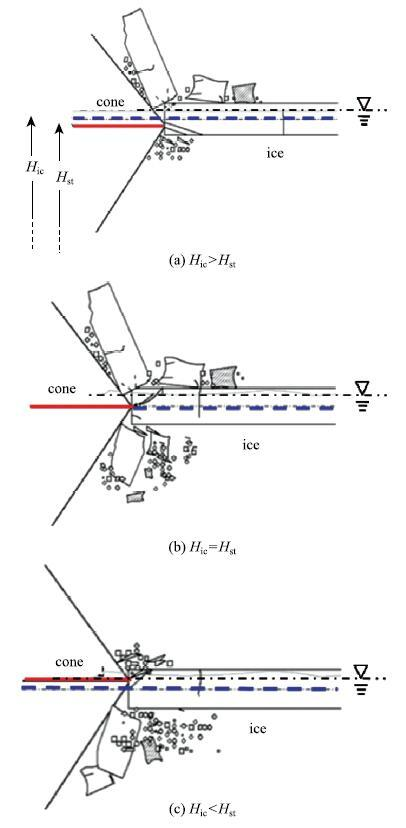Fig.14   Three different contact modes between sea ice and the interface of upward-downward cone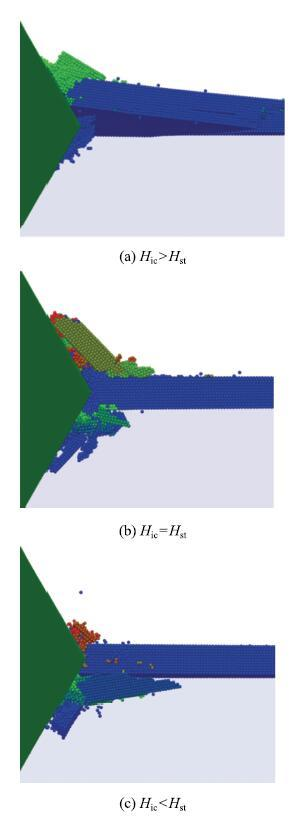Fig.15   Comparison of sea ice damage process in three modes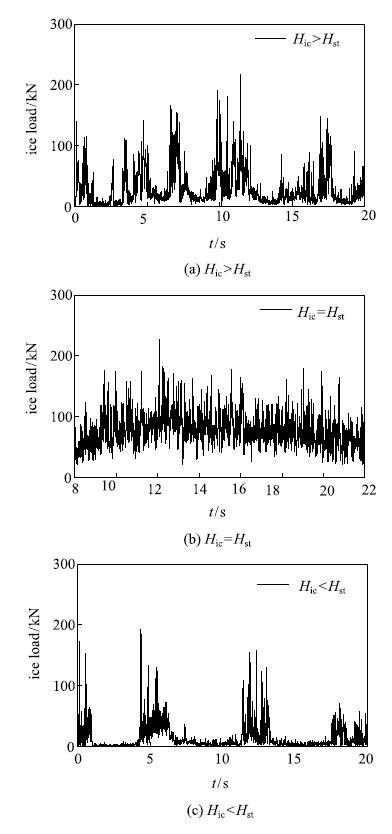Fig.16   Time series of ice loads under three different acting modes

## 4 结论

The authors have declared that no competing interests exist.

## 参考文献 原文顺序 文献年度倒序 文中引用次数倒序 被引期刊影响因子〈〉